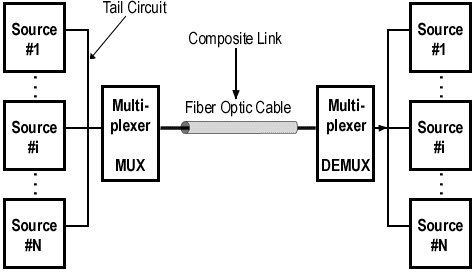Bandwidth utilization is the use of available bandwidth to achieve specific goals. Efficiency can be achieved by using multiplexing; privacy and antijamming can be achieved by using spreading.

Multiplexing is the set of techniques that allows the simultaneous transmission of multiple signals across a single data link. In a multiplexed system, n lines share the bandwidth of one link. The word link refers to the physical path. The word channel refers to the portion of a link that carries a transmission.

There are three basic multiplexing techniques: frequency-division multiplexing, wavelength-division multiplexing, and time-division multiplexing. The first two are techniques designed for analog signals, the third, for digital signals

Frequency-division multiplexing (FDM) is an analog technique that can be applied when the bandwidth of a link (in hertz) is greater than the combined bandwidths of the signals to be transmitted.

Wavelength-division multiplexing (WDM) is designed to use the high bandwidth capability of fiber-optic cable. WDM is an analog multiplexing technique to combine optical signals.

Time-division multiplexing (TDM) is a digital process that allows several connections to share the high bandwidth of a link. TDM is a digital multiplexing technique for combining several low-rate channels into one high-rate one.

We can divide TDM into two different schemes: synchronous or statistical. In synchronous TDM, each input connection has an allotment in the output even if it is not sending data. In statistical TDM, slots are dynamically allocated to improve bandwidth efficiency.

In spread spectrum (SS), we combine signals from different sources to fit into a larger bandwidth. Spread spectrum is designed to be used in wireless applications in which stations must be able to share the medium without interception by an eavesdropper and without being subject to jamming from a malicious intruder.

The frequency hopping spread spectrum (FHSS) technique uses M different carrier frequencies that are modulated by the source signal. At one moment, the signal  modulates one carrier frequency; at the next moment, the signal modulates another carrier frequency.

The direct sequence spread spectrum (DSSS) technique expands the bandwidth of a signal by replacing each data bit with n bits using a spreading code. In other words, each bit is assigned a code of n bits, called chips.